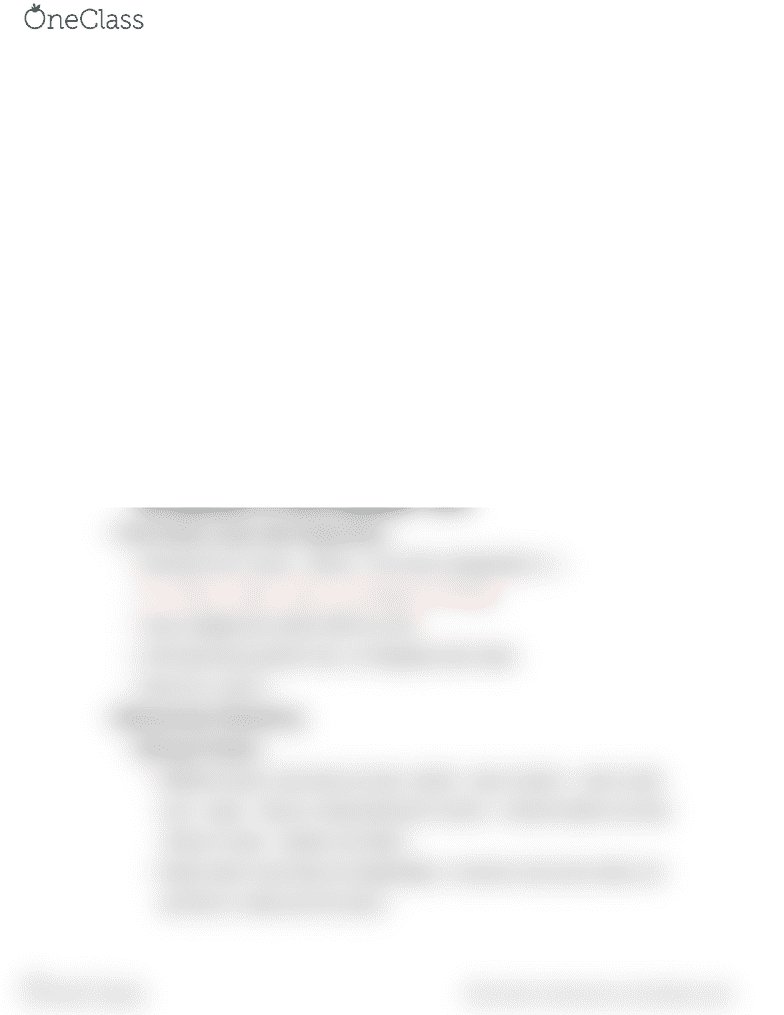# MATH128 Lecture Notes - Lecture 1: Switch, Product Rule

47 views2 pages
School
Department
CourseMath 128 Final Review
Chapter 9
9.1 Ordinary Differential Equations
Linear
Initial Value Problem (provide y, y’ or y^2) can ﬁnd particular solution
Separable ODE:
Particular solution: separate dy and y to the left and dx and x to the right,
then integrate. Make sure to check initial position when x = 0 don't divided
by zero
General solution: cant solve for c, don't need to show y=…
Make sure to check for y=0, if 0=0 then another solution
Use substitution to make a separable (Feb 3)
First Order Linear (Not Separable)
General Form: A(x)y’ + B(x)y = C(x) then manipulate to ->
Y’ + P(X)Y = Q(X), U(X) = e^integral of P(X)dx
Then multiply the whole ODE by U(X)
LHS becomes product rule, so integrate both sides
Solve for y and C
Mathematical Modelling
Mixing Problem
Different rate in and rate out rate. (dx/dt = rate of salt in - rate of salt
out) = (kg/L * ﬂows in rate)-[(amount of salt x / volume-drains out rate
+ﬂows in rate) * (drains out rate)]
Same rate in and rate out (separable), x/volume only since drain out
and ﬂow in rates are the same
find more resources at oneclass.com
find more resources at oneclass.com
Unlock document

This preview shows half of the first page of the document.
Unlock all 2 pages and 3 million more documents.

Already have an account? Log in

# Get access

Grade+
\$10 USD/m
Billed \$120 USD annually
Homework Help
Class Notes
Textbook Notes
40 Verified Answers
Study Guides
1 Booster Class
Class+
\$8 USD/m
Billed \$96 USD annually
Homework Help
Class Notes
Textbook Notes
30 Verified Answers
Study Guides
1 Booster Class